# The Geometric Series

Previous: Convergence of Infinite Series

Next: Geometric Series Example

There are certain forms of infinite series that are frequently encountered in mathematics. The following example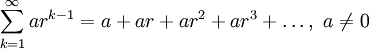for constantsandis known as the geometric series. The convergence of this series is determined by the constant, which is the common ratio.

Theorem: Convergence of the Geometric Series
Letandbe real numbers. Then the geometric series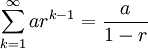converges if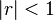, and otherwise diverges.

## Proof

To prove the above theorem and hence develop an understanding the convergence of this infinite series, we will find an expression for the partial sum,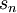, and determine if the limit as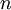tends to infinity exists. We will further break down our analysis into two cases.

### Case 1: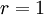If, then the partial sumbecomes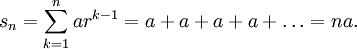So as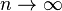we have that.

Hence, the geometric series diverges if r = 1.

### Case 2: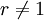A short derivation for a compact expression forwill be useful. First note that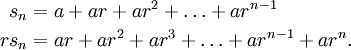The second equation is the first equation multiplied by. Subtracting these two equations yields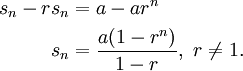Using this result, we see that:

• if, then as n → ∞, sna/(1-r)
• if, then as n → ∞, sn → ∞
• if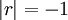, then the series is divergent by the Divergence Test (which we cover in a lesson in Unit 2)

## Summary

The above results can be summarized in the following figure.

Previous: Convergence of Infinite Series

Next: Geometric Series Example